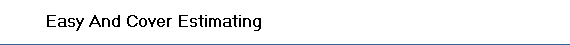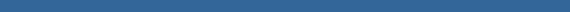##µŃÇÍÂčŇ§ˇŇĂăŞé§ŇąáşşĘ´ : | Beam  | Column | Footing | PierColumn | Slab | Stair | Wall |

### §Ňą°ŇąĂŇˇ• Cut
Distance = Depth/Tan(Slope)
TopWidth = Width+2*(Space+Distance)
TopLength= Length+2*(Space+Distance)
BottomWidth=Width+2*Space
BottomLength=Length+2*Space
BottomArea=BottomWidth*BottomLength
TopArea=TopWidth*TopLength
Cut = (TopArea+BottomArea+Sqr(BottomArea*TopArea))*Depth/3
• SandFill = (Width+2*OverSize)*(Length+2*OverSize)*ThicknessSandFill
• LeanConcrete = (Width+2*OverSize)*(Length+2*OverSize)*ThicknessLeanConcrete
• Concrete = Width*Length*Higth+(Width*Length+0.10+Sqr(Width*Length*0.10)) *HigthTaper/3
• Form = (Width+Length)*2*Higth
• FillBack = Cut-Concrete-SandFill-LeanConcrete
• LowerWidthBar = (Width-FootingCovering*2+2*(Higth-FootingCovering*2)) *NumLowerWidthBar*WeighOfBar
• LowerLengthBar = (Length-FootingCovering*2+2*(Higth-FootingCovering*2)) *NumLowerLengthBar*WeighOfBar
• UpperWidthBar = (Width-FootingCovering*2+2*NumUpperWidthBar*WeighOfBar
• UpperLengthBar =(Length-FootingCovering*2+2*NumUpperLengthBar*WeighOfBar
• HookWidthBar = HookLength*NumLowerWidthBar*WeighOfBar
• HookLengthBar = HookLength *NumLowerLengthBar*WeighOfBar
• StirrupBar = ((Length+Width)*2-FootingCovering*2)*NumStirrupBar*WeighOfBar
• Wire = TotalBar*ConstantWire/1000
• Nail = Form*ConStantNail### PililngCap Footing

• Pililng = NumPile
• Cut = Distance = Depth/Tan(Slope)
TopWidth = Width+2*(Space+Distance)
TopLength= Length+2*(Space+Distance)
BottomWidth=Width+2*Space
BottomLength=Length+2*Space
BottomArea=BottomWidth*BottomLength
TopArea=TopWidth*TopLength
Cut = (TopArea+BottomArea+Sqr(BottomArea*TopArea))*Depth/3
• SandFill = ((Length+2*OverSize)^2)*ThicknessSandFill
• LeanConcrete = ((Length+2*OverSize)^2)*ThicknessLeanConcrete
• Concrete = Length^2*Higth-SectionAreaPile*Lapse
• Form = Length*4*Higth
• FillBack = Cut-Concrete-SandFill-LeanConcrete
• LowerBar = (Length-FootingCovering*2+2*(Higth-Lapse-FootingCovering*2)) *NumLowerBar*WeighOfBar
• SidingBar = (Higth-FootingCovering*2)+2*NumSidingBar*WeighOfBar
• HookLowerBar = HookLength *NumLowerBar*WeighOfBar
• HookSidingBar =HookLength *NumSidingBar*WeighOfBar
• StirrupBar = ((Length*4-FootingCovering*4)*NumStirrupBar*WeighOfBar
• Wire = TotalBar*ConstantWire/1000
• Nail = Form*ConStantNail### Circular Footing

• Pililng = NumPile
• Cut Distance = Depth/Tan(Slope)
BottomArea=3.14159*(Diameter+2*Space)^2/4
TopArea=3.14159*(Diameter+2*(Space+Distant))^2/4
Cut = (TopArea+BottomArea+Sqr(BottomArea*TopArea))*Depth/3
• SandFill = 3.14159*(Diameter+2*OverSize)^2 /4*ThicknessSandFill
• LeanConcrete = 3.14159*(Diameter+2*OverSize)^2 /4*ThicknessLeanConcrete
• Concrete = 3.14159*(Diameter)^2 /4*Higth
• Form = 3.14159*Diameter*Higth
• FillBack = Cut-Concrete-SandFill-LeanConcrete
• LowerBar
AreaFooting = 3.14159*(Diameter-FootingCovering)^2 /4
Length = Sqr(AreaFooting)
LengthBar = Length*NumBar*2
LowerBar = (LengthBar+NumLowerBar*2*(Higth-2*FootingCovering)) *WeighOfBar
• UpperBar
AreaFooting = 3.14159*(Diameter-FootingCovering)^2 /4
Length = Sqr(AreaFooting)
LengthBar = Length*NumBar*2
UpperBar = (LengthBar+NumUpperBar*2*(Higth-2*FootingCovering)) *WeighOfBar
• HookLowerBar = HookLength *NumLowerBar*WeighOfBar
• HookUpperBar = HookLength *NumUpperBar*WeighOfBar
• StirrupBar = 3.14159*Diameter*NumStirrupBar*WeighOfBar
• Wire = TotalBar*ConstantWire/1000
• Nail = Form*ConStantNail### Triangular Footing

• Pililng = NumPile
• Cut Distance = Depth/Tan(Slope)
TopWidth = (Length1+Length2)*0.866+2*(Space+Distance)
TopLength= Length1+Length2+2*(Space+Distance)
BottomWidth=(Length1+Length2)*0.866+2*Space
BottomLength= Length1+Length2+2*Space
BottomArea=BottomWidth*BottomLength
TopArea=TopWidth*TopLength
Cut = (TopArea+BottomArea+Sqr(BottomArea*TopArea))*Depth/3
• SandFill
La1=Length1+2*Length2
La2=La1*0.866
Area=(La1*La2 /2) -(3*Length2^2*0.866/2)
Lo1=2.3094*Space+La1
Lo2=Lo1*0.866
AreaOverSize=(Lo1*Lo2/2)-3*(Length2+1.1547*Space)^2*0.866/2
SandFill= AreaOverSize*ThicknessSandFil
• LeanConcrete = AreaOverSize *ThicknessLeanConcrete
• Concrete = Area*Higth
• Form = (Length1+Higth2)*3*Higth
• FillBack = Cut-Concrete-SandFill-LeanConcrete
• LowerBar = (Sqr(Length1^2+Length^2)-FootingCovering*2+2*(Higth-FootingCovering*2))*

NumLowerBar*WeighOfBar

• HookLowerBar = HookLength *NumLowerBar*WeighOfBar
• StirrupBar = ((Length1+Length2)+3-FootingCovering*3)*NumStirrup*WeighOfBar
• Wire = TotalBar*ConstantWire/1000
• Nail = Form*ConStantNail### Triangular (TwoLayer) Footing

• Pililng = NumPile
• Cut Distance = Depth / Tan(Slope)
TopWidth = Width + 2 * (Space + Distance)
TopLength = Long + 2 * (Space + Distance)
BottomLength = Long + 2 * Space
BottomWidth = Width + 2 * Space
BottomArea = BottomWidth * BottomLength
TopArea = TopWidth * TopLength
Cut = (TopArea + BottomArea + Sqr(BottomArea * TopArea)) * Depth / 3
• SandFill
Area = FWidth * FLong - (FWidth - Length2) / 2 * (FLong - Length1)
AreaOverSize = (Width + OverSize * 2) * (Long + OverSize * 2) - (Width - Length2) / 2 * (Long - Length1)
SandFill= AreaOverSize*ThicknessSandFil
• LeanConcrete = AreaOverSize *ThicknessLeanConcrete
• Concrete = Area*Higth
• Form = (Width + Length1 * 2 + Length2 + 2 * Sqr((Long - Length1) ^ 2 + ((Width - Length2) / 2) ^ 2)) * Higth
• FillBack = Cut-Concrete-SandFill-LeanConcrete
• WidthBar1 = (Width - ConstantFootingCovering * 2 + 2 * (Higth - ConstantFootingCovering * 2)) * WidthBar1 *NumWidthBar1*WeighOfBar
• WidthBar2=(Width - (Width - Length2) / 2 - ConstantFootingCovering * 2 + 2 * (Higth - ConstantFootingCovering * 2)) * NumWidthBar2*WeighOfBar
• LongBar1=(Long - ConstantFootingCovering * 2 + 2 * (Higth - ConstantFootingCovering * 2)) * Num LongBar1*WeighOfBar
• LongBar2= (Long / 2 + Length1 / 2 - ConstantFootingCovering * 2 + 2 * (Higth - ConstantFootingCovering * 2)) * Num LongBar2*WeighOfBar
• HookWidthBar1 = HookLength*NumWidthBar1*WeighOfBar
• HookWidthBar2 = HookLength *NumWidthBar2*WeighOfBar
• HookLongBar1 = HookLength *NumLongBar1*WeighOfBar
• HookLongBar2 = HookLength *NumLongBar2*WeighOfBar
StirrupBar (Width + Length1 * 2 + Length2 + 2 * Sqr((Long - Length1) ^ 2 + ((Width - Length2) / 2) ^ 2)) *NumStirrup*WeighOfBar
• Wire = TotalBar*ConstantWire/1000
• Nail = Form*ConStantNail### Rectangular Footing

• Piling = NumPile
• Cut
Distance = Depth/Tan(Slope)
TopWidth = Width+2*(Space+Distance)
TopLength= Length+2*(Space+Distance)
BottomWidth=Width+2*Space
BottomLength=Length+2*Space
BottomArea=BottomWidth*BottomLength
TopArea=TopWidth*TopLength
Cut = (TopArea+BottomArea+Sqr(BottomArea*TopArea))*Depth/3
• SandFill = (Width+2*OverSize)*(Length+2*OverSize)*ThicknessSandFill
• LeanConcrete = (Width+2*OverSize)*(Length+2*OverSize)*ThicknessLeanConcrete
• Concrete = Width*Length*Higth+(Width*Length+0.10+Sqr(Width*Length*0.10)) *HigthTaper/3
• Form = (Width+Length)*2*Higth
• FillBack = Cut-Concrete-SandFill-LeanConcrete
• LowerWidthBar = (Width-FootingCovering*2+2*(Higth-FootingCovering*2)) *NumLowerWidthBar*WeighOfBar
• LowerLengthBar = (Length-FootingCovering*2+2*(Higth-FootingCovering*2)) *NumLowerLengthBar*WeighOfBar
• UpperWidthBar = (Width-FootingCovering*2+2*NumUpperWidthBar*WeighOfBar
• UpperLengthBar = (Length-FootingCovering*2+2*NumUpperLengthBar*WeighOfBar
• HookWidthBar = HookLength *NumLowerWidthBar*WeighOfBar
• HookLengthBar = HookLength *NumLowerLengthBar*WeighOfBar
• StirrupBar = ((Length+Width)*2-FootingCovering*2)*NumStirrupBar*WeighOfBar
• Wire = TotalBar*ConstantWire/1000
• Nail = Form*ConStantNail### Hexagonal Footing

• Pililng = NumPile
• Cut Distance = Depth/Tan(Slope)
Diameter = Sqr(3)*Length
BottomArea=3.14159*(Diameter+2*Space)^2/4
TopArea=3.14159*(Diameter+2*(Space+Distant))^2/4
Cut = (TopArea+BottomArea+Sqr(BottomArea*TopArea))*Depth/3
• SandFill = 0.866*(Diameter+2*OverSize)^2 *ThicknessSandFill
• LeanConcrete = 0.866*(Diameter+2*OverSize)^2 *ThicknessLeanConcrete
• Concrete = 0.866*(Diameter)^2 /4*Higth
• Form = 6*Length*Higth
• FillBack = Cut-Concrete-SandFill-LeanConcrete
• LowerBar
AreaFooting = 0.866*(Diameter-FootingCovering)^2
Length = Sqr(AreaFooting)
LengthBar = Length*NumBar*2
LowerBar = (LengthBar+NumLowerBar*2*(Higth-2*FootingCovering)) *WeighOfBar
• UpperBar
AreaFooting = 0.866*(Diameter-FootingCovering)^2
Length = Sqr(AreaFooting)
LengthBar = Length*NumBar*2
UpperBar =LengthBar*WeighOfBar
• HookLowerBar = HookLength *NumLowerBar*WeighOfBar
• HookUpperBar = HookLength *NumUpperBar*WeighOfBar
• StirrupBar = LengthBar*NumStirrupBar*WeighOfBar
• Wire = TotalBar*ConstantWire/1000
• Nail = Form*ConStantNail### Hexagonal Footing (2-D Symmetry)

• Pililng = NumPile
• Cut Distance = Depth / Tan(Slope)
BottomArea = (Width1 + Width2 + 2 * Space) * (Length + 2 * Space)
TopArea = (Width1 + Width2 + 2 * (Space + Distance)) * (Length + 2 * (Space + Distance))
Cut = (TopArea + BottomArea + Sqr(BottomArea * TopArea)) * Depth / 3
• SandFill = (Width1 + Width2 + OverSize) * (Length + 2 * OverSize) * ThicknessSandFill
• LeanConcrete = (Width1 + Width2 + OverSize) * (Length + 2 * OverSize) * ThicknessLeanConcrete
• Concrete = (Width1 + Width2) * Length * Higth
• Form = (Sqr((Length / 2) ^ 2 + Width2 ^ 2) * 4 + 2 * Width1) * Higth
• FillBack = Cut - Concrete - SandFill - LeanConcrete
• LowerWidthBar = (Width1 + Width2 + 2 * (Higth - 2 * ConstantFootingCovering)) * NumLowerWidthBar WeighOfBar
• LowerLengthBar = (Length + 2 * (Higth - 2 * ConstantFootingCovering)) * NumLowerLengthBar WeighOfBar
• UpperWidthBar = (Width1 + Width2) * UpperWidthBar * WeighOfBar
• UpperLengthBar = Length * UpperLengthBar* WeighOfBar
• HookLowerWidthBar = HookLength *NumLowerWidthBar*WeighOfBar
• HookLowerLengthBar = HookLength *NumLowerLengthBar*WeighOfBar
• HookUpperWidthBar = HookLength *NumUpperWidthBar*WeighOfBar
• HookUpperLengthBar = HookLength *NumUpperLengthBar*WeighOfBar
• StirrupBar = (Sqr((Length / 2) ^ 2 + Width2 ^ 2) * 4 + 2 * Width1)*NumStirrupBar*WeighOfBar
• Wire = TotalBar*ConstantWire/1000
• Nail = Form*ConStantNail